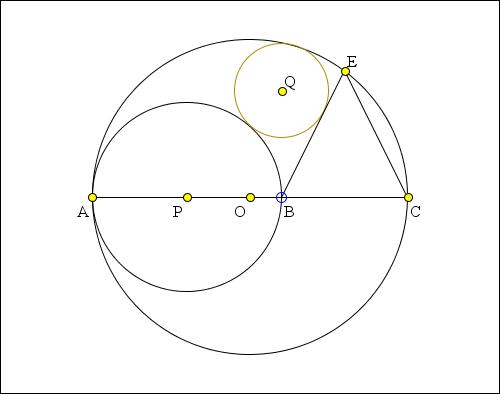### Tangent Circles and an Isosceles Triangle Solution by N. Bowler

The applet presents a solution to the following Sangaku problem: Given a circle S with center O and diameter AC and point B on AC. Form circle G with center P and diameter AB and an isosceles triangle BCE with E on the circle S. Circle W with center Q is inscribed in the curvilinear triangle formed by circles S and G and the line BE. Prove that QB is perpendicular to AC.What if applet does not run? -->

I shall use inversion. I shall denote the inverse of any object by the name of that object followed by a '.

First note that the problem is equivalent to showing that the circle with centre on the perpendicular from B, and tangent to BE and to G is also tangent to S. I shall still call this circle W.

Invert with respect to B, i.e., in a circle centered at B and some radius r, say.1. Step 1: C'BE' will be similar to EBC and so isosceles. This is because BC·BC' = r2 = BE·BE', such that BC/BE = BE'/BC'. It follows that also BC'/C'E' = CE/BE, but in DBCE we have CE = BE.

2. Step 2: S' will be the circle on A'C' as diameter, and G' the tangent to it at A'.

3. Step 3: Let U be the intersection of G' with E'B. Then A'UE' = pi/2 - UBA' = pi/2 - E'BC' = pi/2 - C'E'B = UE'A' so A'UE' is isosceles.

4. Step 4: Let V be the centre of W'. Since W' is tangent to both G' and BE', V lies on the bisector of A'UB. So VUB = A'UV = BVU and so also BVU is isosceles.

5. Step 5: Let L be the centre of S', and call the radius of this circle R. Call the radius of W' r.

There are right angles everywhere, so we may now indulge ourselves with a Pythagorean orgy:

 LV2 = LB2 + BV2 = (R - A'B)2 + BU2 = (R-r)2 + A'B2 + A'U2 = (R-r)2 + r2 + A'E'2 = (R-r)2 + r2 + A'C'2 - E'C'2 = (R-r)2 + r2 + 4R2 - BC'2 = (R-r)2 + r2 + 4R2 - (2R-r)2 = R2 + 2Rr + r2 = (R + r)2.

So that W' is tangent to S' and so W is tangent to S as required.

Another solution makes use of inversion with negative power.### Inversion - Introduction